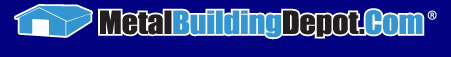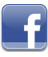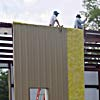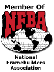888-372-4266
Send an email
MetalBuildingDepot.com
4340 I-10 West, Seguin TX 78155
Open Mon-Fri 8am to 5pmPerformance of InsulationThermal Resistance (R-Value) The measure of the ability of a material to resist heat flow.The higher the R-Value the greater the thermal efficiency.
Overall Heat Transmission Coefficient (U-Value)
A unit used to express heat passage through a complete building section, including air films. U-Values serve as a basis for determining transmitted heat loss. The lower the U-Value, the higher the insulation value.

Calculated Thermal Values
Basically it is the reciprocal of the calculated U-Value. By definition it is the summation of all the R-Values for each of the building's components. This method of calculation does not consider the thermal short circuit effect of structural members, fastener population or the compression of the insulation at its structural members. It should also be noted that this type of calculation often indicates heat loss values for building envelopes that are lower than those actually found in the finished structure.

Thermal Conductivity (K-Value)
A unit used to express the amount of heat, in BTU's per hour, that passes through one square foot of homogeneous material that is exactly one inch thick and has a temperature difference of one degree Fahrenheit between its surfaces. As the K-Value decreases, so does the amount of heat permitted to pass through the material.

Thermal Conductance (C-Value)
A measure of the amount of heat that passes through materials of any thickness.

Surface Air Film Coefficient (F)
The amount of heat flow in BTU's per square foot per hour between an exposed surface of a material and the adjacent air. It is the measure of the conductance of heat through the air film that clings to all surfaces.

AS THE K-VALUE DECREASES, SO DOES THE AMOUNT OF HEAT PERMITTED TO PASS THROUGH THE MATERIAL.Setting the StandardSteel Building BenefitsBenefits of DIYWhy Choose UsFinancingCopyright © 2003-2023 MetalBuildingDepot™. All Rights Reserved. Privacy Policy